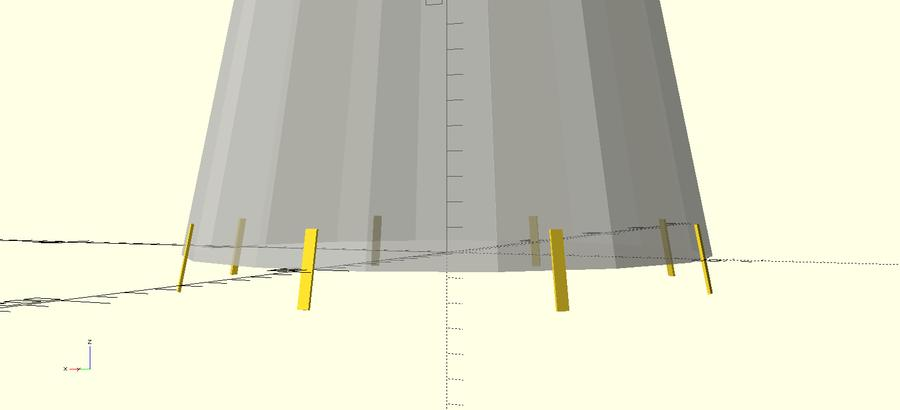# Local coordinate systems in OpenSCADHere's a small snippet for setting a local coordinate system. You need to know the location to treat as origin as well as the direction vectors to treat as X and Y.

```// Take a position vector (p) and 3 direction vectors (uvw)
// and create a transformation matrix.  The origin is transformed to p,
// and xyz point along uvw.
// Normally, the caller will ensure that uvw are orthogonal unit vectors
function puvw(p, u, v, w) = [
[u, v, w, p],
[u, v, w, p],
[u, v, w, p]];

// Take a position vector (p) and 2 direction vectors (uv) which should be
// orthogonal but need not be unit vectors. Calculate the unit vectors as well
// as the third vector and return the resulting matrix
function puv(p, u, v) =
let(nu = u / norm(u), nv = v / norm(v), nw = cross(nu, nv))
puvw(p, nu, nv, nw);

// Operate in a local coordinate system where the origin is the point p,
// x points along u, y points along v, and z points along the normal vector
// to uv. x and y should be orthogonal but need not be unit vectors.
module puv(p, u, v) {
multmatrix(puv(p, u, v)) children();
}
```

For instance, suppose you want to lay out some feature relative to a cone 100mm in diameter and 500mm tall. It's convenient to set the origin to the intersection of the cone base with the +x axis, +x be the direction from the base of the cone to the tip, +y be the direction tangential to the cone, and +z be the direction 'out' from the cone. You might invoke puv like so (corr is a factor to make the flats of the faceted cone exactly touch the 100mm diameter circle):

```effen = 16; // 16, 32, 64, ...
corr = 1 / cos(180/effen);

for(i=[0:45:360]) rotate(i)
puv([100,0,0], [-100, 0, 500], [0, -1, 0])
linear_extrude(height=1)
square([25, 4], center=true);

%rotate(45/4) cylinder(d1=200*corr, d2=0, h=500, \$fn=effen);
```

Entry first conceived on 7 November 2022, 1:35 UTC, last modified on 7 November 2022, 1:52 UTC